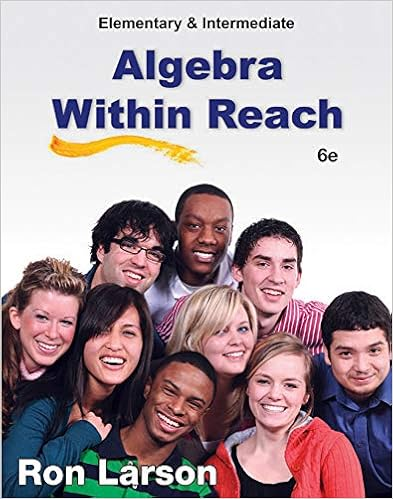# Worksheet-Unit 2(Matriex-and-system equ).pdf - Unit...

• Homework Help
• kunghoyin1995
• 25
• 100% (1) 1 out of 1 people found this document helpful

This preview shows page 1 - 9 out of 25 pages.

##### We have textbook solutions for you!
The document you are viewing contains questions related to this textbook.The document you are viewing contains questions related to this textbook.
Chapter 10 / Exercise 77
Elementary and Intermediate Algebra: Algebra Within Reach
LarsonExpert Verified
Unit 2-WorksheetOpen University of Hong KongMATH S201F Finite Mathematics for BusinessWorksheetUnit 2: Matrices and Systems of Linear equationsThis worksheets contains:Section 1:Basic Operation and Matrix InverseSection 2: Applications of Matrices[You may use Notebook to check you answers.]Section 1:Basic Operation and Matrix InverseInProblems 1°2, the matrices are de°ned as follows:A=°4°3815°7±B=°80°297°3±C=°1°1°2°325±D=24°633°80135O=2400000035Problem 1Verify that the addition of matrices is commutative and associative:1. Verify that the addition of matrices is commutative:A+B=B+A2. Verify that the addition of matrices is associative:A+ (B+C) = (A+B) +CProblem 2Find:1.A+D
Page 1
##### We have textbook solutions for you!
The document you are viewing contains questions related to this textbook.The document you are viewing contains questions related to this textbook.
Chapter 10 / Exercise 77
Elementary and Intermediate Algebra: Algebra Within Reach
LarsonExpert Verified
Unit 2-Worksheet2.DT+B3.AT+O+BT4.D+AT+CTProblem 3LetA=243:87°0:3214:6°9:735:74°2:8011:534:835B=2421:58:86°3:0724:80:894:818:4737:35°6:8335You may use Notebook to check you answers.1.A+BT2.B+ATPage 2
Unit 2-Worksheet3.(A+B)T4.AT+BTInProblems 4°5, the matrices are de°ned as follows:A=266423°4°567533775B=2664°6°308°3°2393775C=°56784321±O=2664000000003775Problem 4Find:1.O°3BPage 3
Unit 2-Worksheet2.B°4A3.CT°2AProblem 5Under the addition of matrix, °nd:1. the additive inverse of(°4C)2. the additive inverse of6A+ 2CT3. the additive matrix of(BT+ 5C)TPage 4
Unit 2-WorksheetInProblems 6°9, the matrices are de°ned as follows:A=°345°4°3°2±B=°3210°1°2±C=242403°1°635D=242°3435E= Problem 6Determine the following matrix product, if any:1.ABT2.3BC3.BC
4. Use Notebook to check the answers obtained in parts 1 to 3.Page 5
Unit 2-WorksheetProblem 7Verify that(AC)B=A(CB):Problem 8Check whether the equalityBTCT=CTBTis valid.Problem 9Find:1.ATB°3DDT2.D±E±CT3. Find the matrixA±D±E:Page 6
Unit 2-Worksheet4. Computing activity: Using Scienti°c Notebook to check your answers obtained in parts 1 to 3.InProblems 10°11, the matrices are de°ned as follows:A=°4°125±B=°°3707±C=D= [°47]Problem 10Find:1. Given that a matrixXsuch thatAX=I. FindX, whereIis the2±2identity matrix.2. Given that a matrixYsuch thatY B=I. FindY.3.det(AB)Page 7
Unit 2-Worksheet4.det(BA)
•••